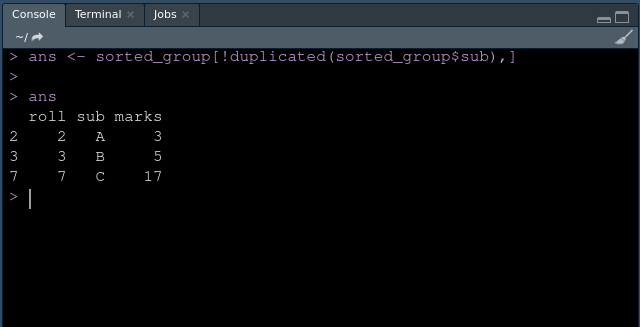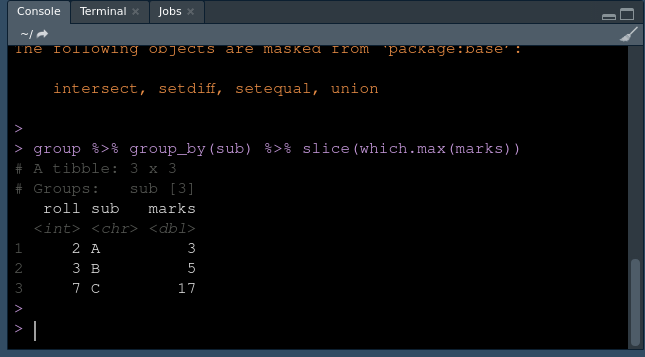Skip to content
Related Articles
How to select row with maximum value in each group in R Language?
• Last Updated : 01 Apr, 2021

In R Programming Language, to select the row with the maximum value in each group from a data frame, we can use various approaches as discussed below.

Consider the following dataset with multiple observations in sub-column. This dataset contains three columns as sr_no, sub, and marks.

Creating Dataset :

Here we are creating dataframe for demonstration.

Code block

Output:

```   roll sub marks
1      1   A     2
2      2   A     3
3      3   B     5
4      4   B     2
5      5   B     5
6      6   C     8
7      7   C    17
8      8   A     3
9      9   C     5
10    10   C     5```

Here, roll and marks are integer value and sub is the categorical value (char) have category A, B, C. In this dataset A, B, C represent different subjects and marks are marks obtained in the corresponding sub.

As we can see subject A, B, C has the maximum value (marks) of 3,5,17 respectively in the group. We can select the max row in the group using the following two approaches.

Methods 1: Using R base.

Step 1: Load the dataset into a variable (group).

## R

 `# Creating a dataset.``no <- ``c``( 1 : 10)``subject <- ``c``(``'A'``, ``'A'``, ``'B'``, ``'B'``, ``'B'``,``             ``'C'``, ``'C'``, ``'A'``, ``'C'``, ``'C'``)`` ` `mark <- ``c``(2, 3, 5, 2, 5, 8, 17, 3, 5, 5)``group <- ``data.frame``(roll = no, sub = subject, ``                    ``marks = mark )`` ` `group`

Output:

```   roll sub marks
1      1   A     2
2      2   A     3
3      3   B     5
4      4   B     2
5      5   B     5
6      6   C     8
7      7   C    17
8      8   A     3
9      9   C     5
10    10   C     5```

Step 2: Sorted the marks in descending order for each group (A, B, C).

## R

 `# Creating a dataset.``no <- ``c``( 1 : 10)``subject <- ``c``(``'A'``, ``'A'``, ``'B'``, ``'B'``, ``'B'``,``             ``'C'``, ``'C'``, ``'A'``, ``'C'``, ``'C'``)``mark <- ``c``(2, 3, 5, 2, 5, 8, 17, 3, 5, 5)``group <- ``data.frame``(roll = no, sub = subject, ``                    ``marks = mark )`` ` `# sorting the sub and marks.``sorted_group <- group[``order``(group\$sub, -group\$marks),]`` ` `sorted_group`

Output:

```   roll sub marks
2      2   A     3
8      8   A     3
1      1   A     2
3      3   B     5
5      5   B     5
4      4   B     2
7      7   C    17
6      6   C     8
9      9   C     5
10    10   C     5```

As our sub is now in ascending order, and we are ready to select the row with max value in each group, here groups are A, B, C.

Step 3: Remove the duplicate rows from the sorted subject column.

## R

 `# Creating a dataset.``no <- ``c``( 1 : 10)``subject <- ``c``(``'A'``, ``'A'``, ``'B'``, ``'B'``, ``'B'``,``             ``'C'``, ``'C'``, ``'A'``, ``'C'``, ``'C'``)``mark <- ``c``(2, 3, 5, 2, 5, 8, 17, 3, 5, 5)`` ` `group <- ``data.frame``(roll = no, sub = subject, ``                    ``marks = mark )`` ` `# sorting the sub and marks.``sorted_group <- group[``order``(group\$sub, -group\$marks),]`` ` `# removing duplicates from the sorted sub column``ans <- sorted_group[!``duplicated``(sorted_group\$sub),]`` ` `ans`

Output:These are the selected row with the maximum value in each group.

Methods 2: Using dplyr package

dplyr is an R package which is most commonly used to manipulate the data frame. dplyr provides various verbs (functions) for data manipulation such as filter, arrange, select, rename, mutate etc.

To install dplyr package we have to run the following command in the R console.

`install.packages("dplyr")`

Step1: Load the dataset and library.

## R

 `# Creating a dataset.``no <- ``c``( 1 : 10)``subject <- ``c``(``'A'``, ``'A'``, ``'B'``, ``'B'``, ``'B'``,``             ``'C'``, ``'C'``, ``'A'``, ``'C'``, ``'C'``)``mark <- ``c``(2, 3, 5, 2, 5, 8, 17, 3, 5, 5)`` ` `group <- ``data.frame``(roll = no, sub = subject, ``                    ``marks = mark )`` ` `# loading library``library``(``"dplyr"``)`

Step 2: Now group the data frame sub using group_ by verb (function) and select the row having maximum marks using which.max().

## R

 `# Creating a dataset.``no <- ``c``( 1 : 10)``subject <- ``c``(``'A'``, ``'A'``, ``'B'``, ``'B'``, ``'B'``,``             ``'C'``, ``'C'``, ``'A'``, ``'C'``, ``'C'``)``mark <- ``c``(2, 3, 5, 2, 5,``          ``8, 17, 3, 5, 5)`` ` `group <- ``data.frame``(roll = no, sub = subject, ``                    ``marks = mark )`` ` `# loading library``library``(``"dplyr"``)`` ` `group %>% ``group_by``(sub) %>% ``slice``(``which.max``(marks))`

Output:As we can see these are the selected row with the maximum value in each group.

My Personal Notes arrow_drop_up